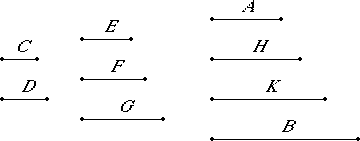# Proposition 12

Between two cubic numbers there are two mean proportional numbers, and the cube has to the cube the triplicate ratio of that which the side has to the side.

Let A and B be cubic numbers, and let C be the side of A, and D of B.

I say that between A and B there are two mean proportional numbers, and A has to K the ratio triplicate of that which C has to D.

Multiply C by itself to make E, and by D to make F, multiply D by itself to make G, and multiply the numbers C and D by F to make H and K respectively.

Now, since A is a cube, and C its side, and C multiplied by itself makes E, therefore C multiplied by itself makes E and multiplied by E makes A. For the same reason also D multiplied by itself makes G and multiplied by G makes B.And, since C multiplied by the numbers C and D makes E and F respectively, therefore C is to D as E is to F. For the same reason also C is to D as F is to G. Again, since C multiplied by the numbers E and F makes A and H respectively, therefore E is to F as A is to H. But E is to F as C is to D. Therefore C is to D as A is to H.

Again, since the numbers C and D multiplied by F make H and K respectively, therefore C is to D as H is to K. Again, since D multiplied by each of the numbers F and G makes K and B respectively, therefore F is to G as K is to B.

But F is to G as C is to D, therefore C is to D as A is to H, as H is to K, and as K is to B.

Therefore H and K are two mean proportionals between A and B.

I say next that A also has to B the ratio triplicate of that which C has to D.

V.Def.10

Since A, H, K, and B are four numbers in proportion, therefore A has to B the ratio triplicate of that which A has to H.

But A is to H as C is to D, therefore A also has to B the ratio triplicate of that which C has to D.

Therefore, Between two cubic numbers there are two mean proportional numbers, and the cube has to the cube the triplicate ratio of that which the side has to the side.

Q.E.D.

## Guide

Between c3 and d3 are two mean proportionals c2d and cd2. The ratio c3 : d3 is the triplicate ratio of c : d.

This proposition is used in VIII.15.